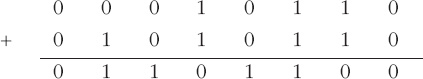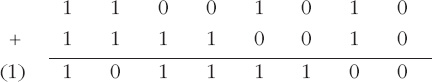Representing and manipulating data in computers

Start this free course now. Just create an account and sign in. Enrol and complete the course for a free statement of participation or digital badge if available.

Free course

# 7.3.2 Subtraction

As I indicated at the start of this section, subtraction is converted to addition by replacing the number to be subtracted by its additive inverse, which in the case of binary arithmetic is its 2's complement. An example should make this clear.

## Example 9

Subtract the signed integer 1010 1010 from the signed integer 0001 0110.

The additive inverse of the number to be subtracted, 1010 1010, is 0101 0101 + 1 = 0101 0110. Using this additive inverse transforms the computation to an addition:(Check: 1010 1010 is −86 and 0001 0110 is 22, so the calculation is 22 − (−86), which is 108, and 0110 1100 is indeed 108.)

## Activity 25 (Self assessment)

Carry out the following subtraction by first finding the additive inverse of the number to be subtracted:

1100 1010 − 0000 1110

The additive inverse of the integer to be subtracted is its complement plus 1:

1111 0001 + 0000 0001

which is 1111 0010.

So the calculation is now:The ninth bit can be ignored here, as mentioned in Section 7.2. So the result is 1011 1100.